# Synthesis and Analysis of a Ferric Oxalate Salt

By: Omish Samaroo

Introduction

The purpose of this lab is as follows: 1) to learn about coordination compounds through the synthesis and analysis of a potassium ferrioxalate hydrate salt and 2) to use the techniques developed in previous labs to determine the empirical formula of the ferrioxalate salt. The ferrioxalate salt has the empirical formula Kx[Fey(C2O4)z]•nH2O. The values of x, y, z, and n are ascertained through determining the percentages of K, Fe, C­2O42-, and H2O; converting these percentages to grams and then grams to moles; and using mole ratios to attain the values for each variable.

The following is the balanced chemical reaction equation for the formation of the potassium ferrioxalate salt:

FeCl3•6H2O (aq) + 3K2C2O4•H2O (aq) àK3Fe(C2O4)3•3H2O (s) + 3KCl (aq) + 6H2O (l)

In the potassium ferrioxalate hydrate salt, the central atom is Iron, Fe, which is in the 3+ oxidation state. The Fe3+ ion, also called the ferric ion, can have 6 ligands, which act as Lewis bases in coordinate covalent bonds. Coordinate covalent bonds, sometimes referred to as dative bonds, is different in the sense that one atom donates both electrons to form a pair of electrons which is shared between two atoms while the other atom doesn’t donate any electrons to this bond, as opposed to an ordinary covalent bond where each atom donates a valence electron to form a pair of electrons which is shared between the two atoms. All dative bonds result from Lewis acid/base reactions. Some ligands, called chelates, have more than one pair of electrons which they can donate to the central atom. Compound which contain dative bonds are called coordination compounds. The coordination number of a coordination compound is the number of sites that ligands are attached to the metal. The ferric ion can have 6 ligands; therefore, its coordination number is 6, and thus has an octahedral geometry.

Once the complex salt potassium ferrioxalate hydrate is synthesized and filtered, the percentages of K, Fe, C2O42-, and H2O can be calculated. The percentage of H2O is calculated by taking a specific amount of the solid and heating it to evaporate the water, calculating the mass of H2O loss, and calculating the %H2O by mass:

(mass of H2O loss/ mass of solid used) x 100% = %H2O by mass

The percentage of C2O42- is calculated through redox titration using potassium permanganate, KMnO4. First, the concentration has to be standardized using sodium oxalate, Na2C2O4. The molarity of permanganate solution is used, along with a mole ratio from the balanced net ionic equation of the reaction of potassium permanganate with sodium oxalate to calculate the grams of oxalate, which can then be used to calculate the %C2O42-. The balanced net ionic reaction is as follows:

2MnO4(aq) + 5C2O42- (aq) + 16H+ (aq) à 2Mn2+ (aq) + 10CO2 (g) + 8H2O (l)

The percentage of Fe is calculated through colorimetric analysis. This involves serial dilutions of an Fe stock solution at different volumes, using a spectrometer to determine the molar absorptivity of the stock solution and to determine the absorptivity of a solution of ferrioxalate salt, using these to calculate the amount of moles and grams of Fe, and using this value to calculate the %Fe. The following equations are essential for this part:

A = 2- log(%T) à %T = 102-A,

where A is the absorbance, which the number received from the spectrometer readings, and %T is the percent transmittance;

A = Ԑ*l*C,

where A is the absorbance, Ԑ is the molar absorptivity ( in units of M-1cm-1), l is the path length (which is equal to 1cm), and C is the concentration (in moles per liter, or M);

Concentration of the stock solution= 50(concentration of the diluted solution;

and

M1V1=M2V2,

where M1 is the initial concentration, V1 is the initial volume used, M2 is the concentration after dilution, and V2 is the volume of the solution used in the dilution.

Finally, the percentage of K is calculated by difference, using the percentages of water, oxalate, and iron:

%K = 100% – (%H2O + %C2O42- + %Fe)

As stated before, these percentages are then used to calculate the values for x, y, z, and n in the empirical formula, Kx[Fey(C2O4)z]•nH2O. When the empirical formula is determined, percent error is ascertained by using the molar mass of the determined empirical formula and the theoretical molar mass of the salt:The theoretical yield is calculated by using stoichiometric ratios to determine the amount of ferrioxalate that would theoretically be produced from the exact amounts of iron (III) chloride hexahydrate and potassium oxalate that were measured from the beginning of the experiment. The percent yield is then determined by the following equation:

% Yield = (actual yield/ theoretical yield) * 100%

Experimental Method

Part 1: Synthesis of the Potassium Ferrioxalate Salt

An exact mass of 6.0556 g of iron (III) chloride hexahydrate was obtained, placed into a 50-mL beaker, and mixed with 10 mL of distilled water. Then, an exact mass of 13.6140 g of potassium oxalate was obtained, placed into a 100-mL beaker, and mixed with 22 ml of distilled water. Both beakers were placed onto a hot plate and heated while being stirred until the salts were completely dissolved. (Note: the solutions were not heated to boiling.) The hot iron solution was then added to the hot oxalate solution and stirred. The beaker containing the solution was placed onto a wired gauze pad and left to cool to room temperature. While the solution was being cooled, an ice-water bath was prepared. The beaker was then placed into the ice-water bath until no further crystallization occurred. The mother liquor in the beaker was decanted from the crystals, leaving the crystals at the bottom of the beaker. Then, 20 ml of distilled water was added to the beaker and heated to dissolve the crystals. The beaker was cooled and then placed into the ice-water bath until no further crystallization occurred. While the solution was being cooled, a Büchner funnel fitted with filter paper was set up for vacuum filtration. The crystals were placed into the Büchner funnel and the vacuum filter was turned on. When the vacuum filter was turned off, the crystals were covered with 50% (v:v) ethanol/water solution. The vacuum was then turned on to remove the solution from the crystals. The vacuum was turned off and the crystals were then washed with acetone. A paper towel was placed over the funnel to protect the crystals from light. The vacuum was turned on for 10 minutes. The crystals were transferred to a clean, dry watch glass, covered with a paper towel, and stored in a  drawer to be analyzed during the following weeks.

Part 2: Analysis of the Potassium Ferrioxalate Salt

A blue weighing boat was placed onto the analytical balance and zeroed. The crystals were transferred to the weighing boat and the mass was recorded. The crystals were then crushed using a mortar and pestle. The crystals were transferred into a large test tube with a stopper. The outside of the test tube was covered with aluminum foil to protect the crystals from light.

The masses of two distinct crucibles were obtained on the analytical balance. While the smaller crucible was on the balance, an exact mass of 0.8023 g of the ferrioxalate crystal was transferred into the crucible; while the larger crucible was on the balance, an exact mass of 0.8042 g of the salt was transferred into the crucible. (Note: tongs were used to prevent the transfer of finger oils onto the crucibles.) The crucibles were placed into a beaker and then placed into an oven at 110° C for one and a half hours.

While the crystals were being heated, the buret was cleaned and conditioned with potassium permanganate, and the buret apparatus was set up. A blue weighing boat was placed onto the analytical balanced and zeroed. An exact mass of 0.252 g of sodium oxalate was transferred to the weighing boat. The sodium oxalate was then transferred into a 250-mL Erlenmeyer flask, using 50 mL of distilled water to wash all of solid into the flask. The flask was swirled to dissolve the solid. Carefully, 10 mL of 4 M H2SO4 was added to the flask. The flask was then heated on a hot plate until the solution was at 80°C. While the solution was being heated, the buret was filled with potassium permanganate to the 0.00 mL line. The flask was removed from the hot plate and the titration process then began. This process was done a second time using an exact mass of 0.253 g of sodium oxalate instead. The average molarity was then calculated. An exact mass of 0.0829 g of the ferrioxalate salt was measured, placed into an Erlenmeyer flask with 50 ml of distilled water and 10 mL of 4 M H2SO4, heated on the hot plate to 80°C, and titrated using potassium permanganate. The same was done with an exact mass of 0.0835 g of the ferrioxalate salt. The percentage of C2O42- was calculated.

After the titration process was complete and one and a half hours passed, the beaker containing the two crucibles were removed from the oven. The crucibles were taken out using tongs and placed onto a wire gauze pad to cool for five minutes. Once cooled, the mass of the two crucibles containing the ferrioxalate anhydrous were measured. The percentage of H2O by mass was calculated.

Results

Mass of FeCl3•6H2O = 6.0556 g

Mass of K2C2O4•H2O = 13.6140 g

Exact mass of crystals (actual yield) = 8.917 g

% H2O

Table 1. The measurements and calculations necessary to calculate the % H2O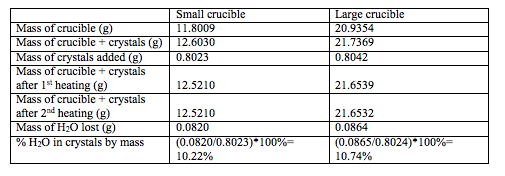Average % H2O by mass = (10.22+12.74)/2 = 10.48% H2O

Uncertainty: m(bar)= 10.5% ± 0.4%

% C2O42-

Table 2. The measurements and calculations necessary to calculate the molarity of the KMnO4 standard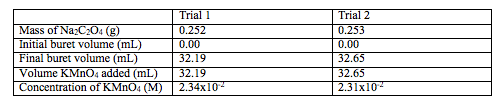Average molarity of KMnO = [(2.34×10-2)+( 2.31×10-2)]/2 = 2.33×10-2

Uncertainty: m(bar)= 2.0×10-2 M ± 2.0×10-4 M

Table 3. The measurements and calculations necessary to calculate % C2O42-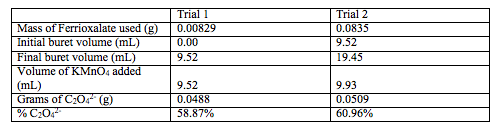Average % C2O42- = (58.87+60.96)/2 = 59.92% C2O42-

Uncertainty: m(bar) = 59.9 % ± 1.5 %

% Fe

Mass of ferrous ammonium sulfate hexahydrate = 0.4904 g

Concentration of stock solution= 5.05×10-2 M

Table 4. The concentration of each solution after diluting (by taking out 5 mL of each solution and filling it to the line with distilled water and using M1V1=M2V2 to calculate the second concentration)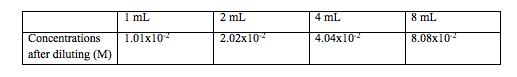Table 5. Shows the spectrometer readings and the % transmittance for each solution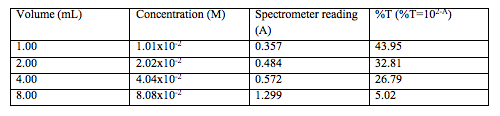Graph 1. Shows the Absorbance vs. Concentration and a line of best fit for the data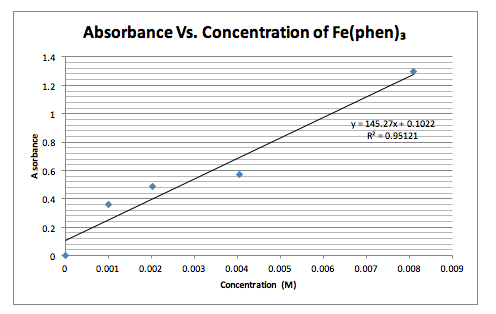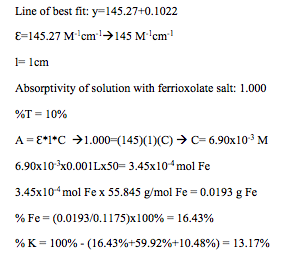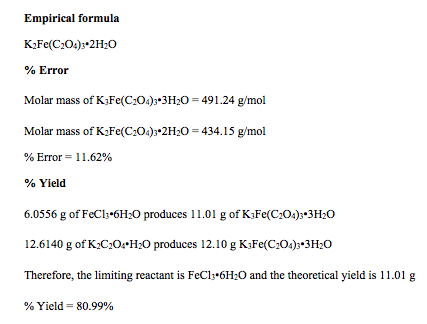Discussion

The actual empirical formula of potassium ferrioxalate is K3Fe(C2O4)3•3H2O, which has a molar mass of 491.24 g/mol. The IUPAC name for this salt is potassium iron(III) oxalate trihydrate. In this experiment, an empirical formula of K2Fe(C2O4)3•2H2O, which has a molar mass of 434.15 g/mol, was ascertained. Therefore, there was a percent error of 11.62%. With 6.0556 g of FeCl3•6H2O and 12.6140 g of K2C2O4•H2O, since FeCl3•6H2O is the limiting reactant in this experiment, there is a theoretical yield of 11.01 g, which means that there was an 80.99% yield in this experiment. This is a really high percent yield. Possible errors include some of the crystal being left on whatever surface it left in its many transfers, some of the crystal being misplaced from the Büchner funnel when being transferred to the watch glass, and too much light being exposed to the crystals, which causes decomposition of this particular compound.

Figure 1 shows the structure of the potassium ferrioxalate. The oxygens, which are single bonded to carbon, are the ones that bond to the iron. The overall charge on complex ferrioxalate ion is 3- because Fe is 3+ and there three oxalate ions each with a charge of 2-, which this result in a total charge of 6- for the oxalate ion. The 3- charge on the complex ion is compensated by three 1+ potassium ions. The potassium ions are on the outside of the complex ion.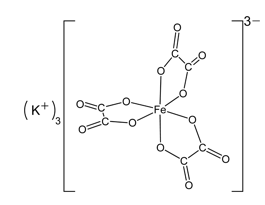Table 6. Shows the observed percent composition, the correct composition, and the percent error for each composition of the ferrioxalate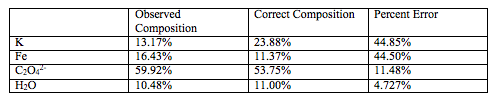Possible errors include some of the crystal being left on whatever surface it left in its many transfers, some of the crystal being misplaced from the Büchner funnel when being transferred to the watch glass, and too much light being exposed to the crystals, which causes decomposition of this particular compound.

References

Mills, B. (2008, March 30). Potassium Ferrioxalate. Wikipedia. Retrieved November 6, 2012, from en.wikipedia.org/wiki/File:Potassium-ferrioxalate-2D.png

Potassium ferrioxalate – Wikipedia, the free encyclopedia. (n.d.). Wikipedia, the free encyclopedia. Retrieved November 6, 2012, from http://en.wikipedia.org/wiki/Potassium_ferrioxalate

• Share